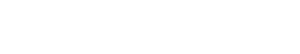# Linear

Linear has a specific meaning in mathematics and statistics. In these fields, Linear refers to a straight line on a graph. This usage is related to the text analytics usage, in that both refer to a way of representing data. However, the specific meaning of Linear in mathematics and statistics is different from the meaning in text analytics.

## Linear Classifiers

A linear classifier is a machine learning algorithm that makes predictions based on a linear combination of the input features. In other words, it predicts using a line (or hyperplane) that best separates the classes. For example, a linear classifier could be used to separate emails into spam and non-spam.

Linear classifiers are often used as a baseline model before trying more complex models. They are also used when the number of features is large, or when the data is not linearly separable.

Linear classifiers are fast and easy to train, but they can only learn linear decision boundaries. This means that they might not be able to accurately classify points that are not on a straight line.

## Features and Weight as Linear Classifiers

The inputs to a linear classifier are called features, and each feature has a corresponding weight. The weights represent the importance of each feature in the decision boundary. For example, if the first feature is the amount of money spent on a product, and the second feature is the number of reviews for the product, then a high weight for the first feature means that money is a more important factor than reviews.

A linear classifier predicts the class of input by computing the dot product of the input features and the weights and then applying a threshold. If the dot product is greater than the threshold, the class is predicted to be positive (1), otherwise, it is predicted to be negative (0).

The threshold is a parameter of the linear classifier that can be tuned to get the best performance.

## Bag of Words as Linear Classifiers

A bag of words is a representation of text data that ignores grammar and order. Each word is represented by a number, and the bag of words representation is simply a vector of those numbers.

For example, consider the following two sentences:

The cat sat on the mat.

The dog slept on the bed.

A bag of words representation for these two sentences would be:

(2, 1, 0, 1, 1)

(1, 0, 1, 1, 0)

## Naive Bayes as Linear Classifiers

Naive Bayes is a type of linear classifier. It makes predictions based on the probability of each input feature belonging to each class. For example, if the first feature is the amount of money spent on a product, and the second feature is the number of reviews for the product, then a high weight for the first feature means that money is a more important factor than reviews.

Naive Bayes is a fast and easy to train, but it can only learn linear decision boundaries.Unlock the power of actionable insights with AI-based natural language processing.